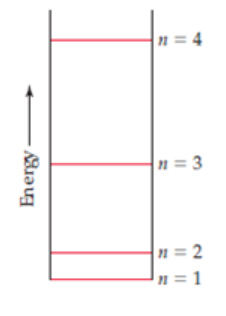# Problem: A certain quantum mechanical system has the energy levels shown in the accompanying diagram. The energy levels are indexed by a single quantum number n that is an integer, (a) As drawn, which quantum numbers are involved in the transition that requires the most energy?(b) Which quantum numbers are involved in the transition that requires the least energy?(c) Based on the drawing, put the following in order of increasing wavelength of the light absorbed or emitted during the transition: (i) n = 1 to n = 2(ii) n = 3 to n = 2(iii) n = 2 to n = 4(iv) n = 3 to n = 1

###### FREE Expert Solution

↑ E → ↓ λ

Based on the diagram, the order of increasing energy of the given transitions are as follow###### Problem Details

A certain quantum mechanical system has the energy levels shown in the accompanying diagram. The energy levels are indexed by a single quantum number n that is an integer,

(a) As drawn, which quantum numbers are involved in the transition that requires the most energy?

(b) Which quantum numbers are involved in the transition that requires the least energy?

(c) Based on the drawing, put the following in order of increasing wavelength of the light absorbed or emitted during the transition:

(i) n = 1 to n = 2

(ii) n = 3 to n = 2

(iii) n = 2 to n = 4

(iv) n = 3 to n = 1